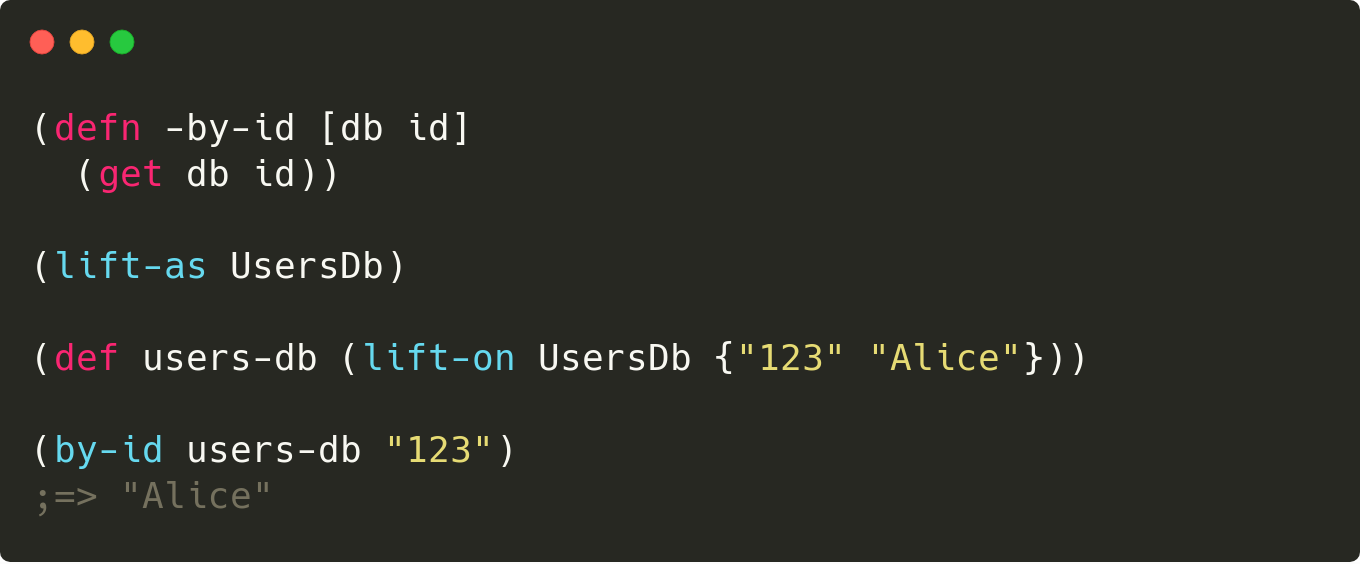Liking cljdoc? Tell your friends :D

# 🚡 Lifted

A small Clojure library for lifting functions into protocols. It allows an easy way to protocolize a (default) implementation, which can be useful for testing (mocking) purposes for example.## Usage

The library offers two macros and a function.

``````(require '[lifted.core :refer [lift-as lift-on lifted]])
``````

The `lift-as` macro declares a protocol. Its sole argument is the name of the protocol to declare. The macro "lifts" the functions in the current namespace which names are prefixed with the `-` character into the protocol. The prefix is stripped from the protocol function names. Only those functions which take at least one argument are lifted.

For example, let's create a simple prefixed function:

``````(defn -incr
"Incrementer function."
([this] (-incr this 1))
([this n] (+ this n)))

(lift-as Incrementer)
``````

The `lift-as` macro above expands to:

``````(defprotocol Incrementer
(incr [this] [this n]
"Incrementer function."))
``````

The `lift-on` macro creates a protocol implementation. Its two arguments are the protocol to implement and the object to use as `this`. The protocol simply forwards the calls to the prefixed functions, passing the `this` object as the first argument. For example:

``````(def i (lift-on Incrementer 5))
``````

The `lift-on` macro above expands to:

``````(def i
(let [G__3427 5]
(reify Incrementer
(incr [this] (-incr G__3427))
(incr [this n] (-incr G__3427 n))

lifted.core/Lifted
(lifted [_] G__3427)))
``````

Now you can use the protocol implementation as you would with any protocol.

``````(incr i)
;=> 6

(incr i 10)
;=> 15
``````

To retrieve the object on which the protocol implementation was lifted on, you can use the `lifted` function. For example:

``````(lifted i)
;=> 5
``````

### Closing remarks

#### Varargs

Clojure protocols do not support varargs. Therefore the lifted function argument lists with a vararg are normally stripped from the protocol. If however one needs varargs, you can pass an option map to `lift-as` as follows:

``````(defn -vararg-test [y & ys]
...)

(lift-as VarargTest {:expand-varargs-for #{vararg-test}})
``````

This will expand the vararg into concrete arguments, up to a maximum of 20 total arguments. For example, above `lift-as` macro would expand to:

``````(defprotocol VarargTest
(vararg-test [y] [y ys_0] [y ys_0 ys_1] . . . [y ys_0 ys_1 .. ys_18]))
``````

The rule still applies that for a function to be lifted it should at least take one argument.

#### Destructuring

The macros do not support destructuring syntax in the signatures of the to-be lifted functions. Please do the destructuring inside the function, using a `let` for example.

That's it. Enjoy! 🚀